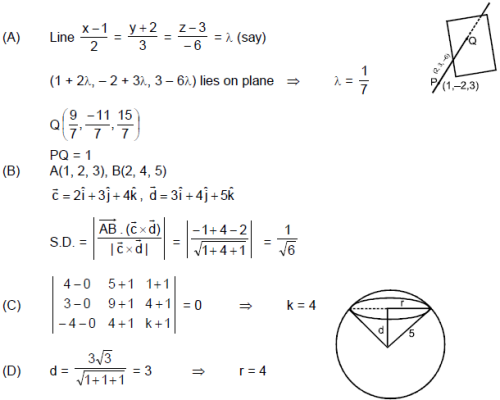Courses

# Part Test - 12 (JEE Advanced 2021)

## 72 Questions MCQ Test National Level Test Series for JEE Advanced 2020 | Part Test - 12 (JEE Advanced 2021)

Description
This mock test of Part Test - 12 (JEE Advanced 2021) for JEE helps you for every JEE entrance exam. This contains 72 Multiple Choice Questions for JEE Part Test - 12 (JEE Advanced 2021) (mcq) to study with solutions a complete question bank. The solved questions answers in this Part Test - 12 (JEE Advanced 2021) quiz give you a good mix of easy questions and tough questions. JEE students definitely take this Part Test - 12 (JEE Advanced 2021) exercise for a better result in the exam. You can find other Part Test - 12 (JEE Advanced 2021) extra questions, long questions & short questions for JEE on EduRev as well by searching above.
QUESTION: 1

### A cobalt (atomic no. = 27) target is bombarded with electrons, and the wavelengths of its characteristic x-ray spectrum are measured. A second weak characteristic spectrum is also found, due to an impurity in the target. The wavelengths of the Kα lines are 225.0 pm (cobalt) and 100.0 pm (impurity).  Atomic number of the impurity is (take b = 1)

Solution: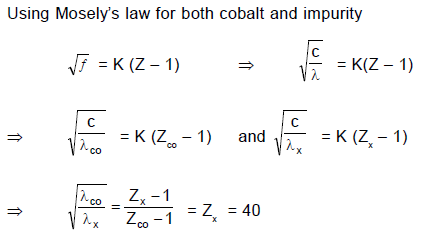QUESTION: 2

### A hydrogen atom is in the 4th excited state, then:

Solution:

The hydrogen atom is in n = 5 state.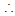Max. no of possible photons = 4
To emit photon in ultra violet region, it must jump to n = 1, because only Lyman series lies in u.v. region. Once
it jumps to n = 1 photon, it reaches to its ground state and no more photons can be emitted. So only one photon
in u. v. range can be emitted.
If H atom emits a photon and then another photon of Balmer series, option D will be correct.

QUESTION: 3

### In the photoelectric experiment, if we use a monochromatic light, the I - V curve is as shown. If work function of the metal is 2eV, estimate the power of light used. (Assume efficiency of photo emission = 10–3%, i.e. number of photoelectrons emitted are 10–3% of number of photons incident on metal.)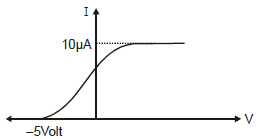Solution: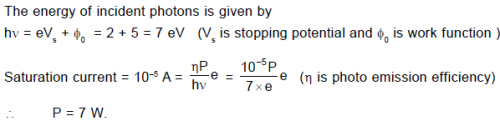QUESTION: 4

In the following question, a Statement of Assertion (A) is given followed by a corresponding Reason(R) just below it.Read the Statement carefully and mark the correct answer-
Assertion(A): Protostele is the simplest type of stele.
Reason (R): Siphonostele has pith in the centre.

Solution:
QUESTION: 5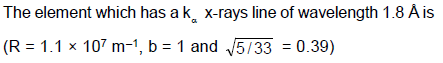Solution: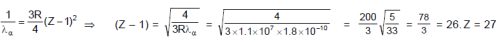QUESTION: 6

A 10 μ F capacitor and a 20 μ F capacitor are connected in series across 200 V supply line. The charged capacitors are then disconnected from the line and reconnected with their positive plates together and negative plates together and no external voltage is applied. What is the potential difference across each capacitor?

Solution:
QUESTION: 7

A metallic charged ring is placed in a uniform magnetic field with its plane perpendicular to the field. If the magnitude of field starts increasing with time, then :

Solution: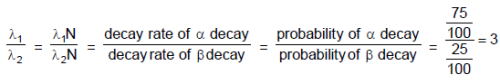QUESTION: 8

An electron in hydrogen atom makes a transition n1 → n2 where n1 and n2 are principal quantum numbers of the two states. Assuming Bohr's model to be valid the time period of the electron in the initial state is eight times that in the final state. The possible values of n1 and n2 are

Solution:
*Multiple options can be correct
QUESTION: 9

The decay constant of a radio active substance is 0.173 (years)-1. Therefore :

Solution: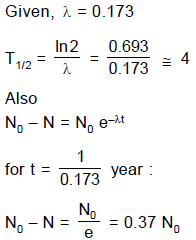*Multiple options can be correct
QUESTION: 10

In an x-ray tube the voltage applied is 20 kV. The energy required to remove an electron from L shell is 19.9 keV. In the x-rays emitted by the tube (Use hc = 12420 eVÅ)

Solution: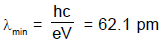*Multiple options can be correct
QUESTION: 11

When a hydrogen atom  is excited from ground  state to first excited state then

Solution: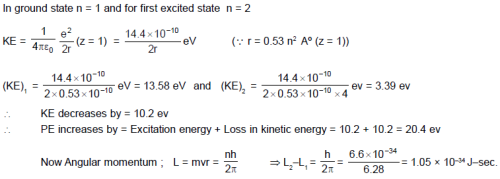QUESTION: 12

Consider the following four electrodes,

P = Cu2+ (0.0001 M) | Cu(s)

Q = Cu2+ (0.1 M) | Cu(s)

R = Cu2+ (0.01 M) | Cu(s)

S = Cu2+ (0.001 M) | Cu(s)

If the standard reduction potential of Cu2+/Cu is +0.34 V, the reduction potentials in volts of the above electrodes follow the order

Solution:
QUESTION: 13

Statement-1 : In process of photoelectric emission, all emitted electrons donot have same kinetic energy.

Statement-2 : If radiation falling on photosensitive surface of a metal consists of different wavelengths, then energy acquired by electrons absorbing photons of different wavelengths shall be different.

Solution:

Both statements I and II are true; but even if radiation of single wavelength is incident on photosensitive surface, electrons of different KE will be emitted.

QUESTION: 14

Statement-1 : When a beam of highly energetic neutrons is incident on a tungsten target, no X-rays will be produced.

Statement-2 : Neutrons do not exert any electrostatic force on electrons or nucleus of an atom.

Solution:

Neutron will knock out electrons in target leading to production of characteristic X-ray. Hence statement-1 is false.

QUESTION: 15

Statement-1 : When cathode rays (accelerated with high voltage 10 kVolts) strikes a hard metallic surface, highly penetrating radiation is obtained.

Statement-2 : Due to conversion of electron's kinetic energy into photon's energy, the shortest wavelength limit of X-rays produced is inversely proportional to the accelerating voltage.

Solution: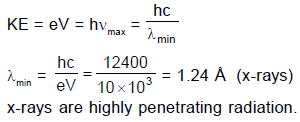QUESTION: 16

Statement-1 : Heavy nuclides tend to have more number of neutrons than protons.

Statement-2 : In heavy nuclei, as there is coloumbic repulsion between protons, so excess of neutrons are preferable.

Solution:

Statement-1 is True, Statement-2 is True; Statement-2 is a correct explanation for Statement-1

QUESTION: 17

An experimental setup of verification of photoelectric effect is shown in the diagram. The voltage across the electrodes is measured with the help of an ideal voltmeter and which can be varied by moving jockey ‘J’ on the potentiometer wire. The battery used in potentiometer circuit is of 20 V and its internal resistance is 2?. The resistance of 100 cm long potentiometer wire is 8?.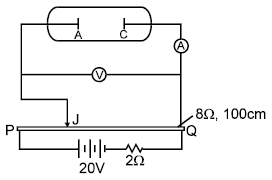The photocurrent is measured with the help of an ideal ammeter. Two plates of potassium oxide of area 50 cm2 at separation 0.5 mm are used in the vacuum tube. Photo current in the circuit is very small so we can treat potentiometer circuit an independent circuit.

The wavelengths of various colours is as follows :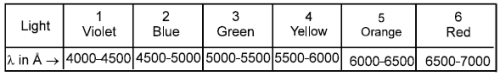Q. The number of electrons appeared on the surface of the cathode plate, when the jockey is connected at the end ‘P’ of the potentiometer wire. Assume that no radiation is falling on the plates.

Solution: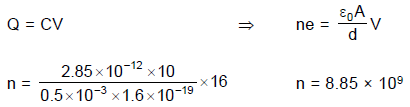QUESTION: 18

An experimental setup of verification of photoelectric effect is shown in the diagram. The voltage across the electrodes is measured with the help of an ideal voltmeter and which can be varied by moving jockey ‘J’ on the potentiometer wire. The battery used in potentiometer circuit is of 20 V and its internal resistance is 2?. The resistance of 100 cm long potentiometer wire is 8?.The photocurrent is measured with the help of an ideal ammeter. Two plates of potassium oxide of area 50 cm2 at separation 0.5 mm are used in the vacuum tube. Photo current in the circuit is very small so we can treat potentiometer circuit an independent circuit.

The wavelengths of various colours is as follows :Q. It is found that ammeter current remains unchanged (2μA) even when the jockey is moved from the end ‘P’ to the middle point of the potentiometer wire. Assuming all the incident photons eject electron and the power of the light incident is 4 × 10–6 W. Then the colour of the incident light is

Solution: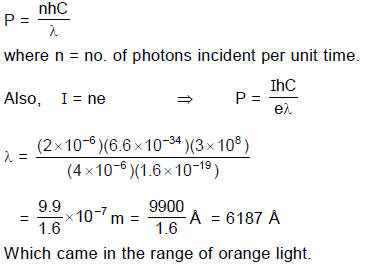QUESTION: 19

An experimental setup of verification of photoelectric effect is shown in the diagram. The voltage across the electrodes is measured with the help of an ideal voltmeter and which can be varied by moving jockey ‘J’ on the potentiometer wire. The battery used in potentiometer circuit is of 20 V and its internal resistance is 2?. The resistance of 100 cm long potentiometer wire is 8?.The photocurrent is measured with the help of an ideal ammeter. Two plates of potassium oxide of area 50 cm2 at separation 0.5 mm are used in the vacuum tube. Photo current in the circuit is very small so we can treat potentiometer circuit an independent circuit.

The wavelengths of various colours is as follows :Q. When other light falls on the anode plate the ammeter reading remains zero till,  jockey is moved from the end P to the middle point of the wire PQ. Thereafter the deflection is recorded in the ammeter. The maximum kinetic energy of the emitted electron is

Solution: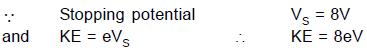QUESTION: 20

Consider the reaction,

Cl2 (aq) + H2S (aq) → S(s) + 2H+(aq) + 2Cl(aq)

The rate equation for this reaction is, rate = k [Cl2] [H2S]

Which of these mechanisms is/are consistent with this rate equation?

(a).  Cl2 + H2S → H+ + Cl + Cl+ + HS (slow)

Cl+ + HS → H+ + Cl + S (fast)

(b).  H2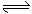H+ + HS (fast equilibrium)

Cl2 + HS → 2Cl + H+ + S (slow)

Solution:
QUESTION: 21

The half life period of a first order chemical reaction is 6.93 minutes. The time required for the completion of 99% of the chemical reaction will be (log 2 = 0.301)

Solution:
QUESTION: 22

Consider a reaction, aG + bH → Products.

When concentration of both the reactants G and H is doubled, the rate increases by eight times. However, when the concentration of G is doubled keeping the concentration of H fixed, the rate is doubled. The overall order of the reaction is:

Solution:
QUESTION: 23

Using Bohr’s model for H–like atom, match the following. Here n = orbit number, Z = nuclear charge, m = mass of electron
Column-I                                                                              Column-II
(A) Due to revolving electron, the magnetic field produced                (p) n–5
at its centre is proportional to

(B) Magnetic moment of revolving electron is proportional to             (q) n

(C) De-Broglie wave length of revolving electron is proportional to      (r) Z3

(D) Areal velocity of revolving electron about nucleus is                    (s) independent of Z
proportional to
(t) m–1

Solution: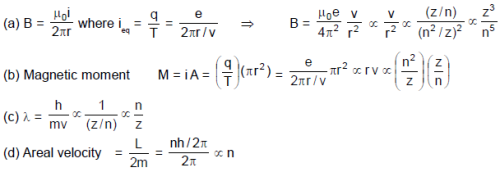QUESTION: 24

For a reaction A + B → C + D if the concentration of A is doubled without altering the concentration of B, the rate gets doubled. If the concentration of B is increased by nine times without altering the concentration of A, the rate gets tripled. The order of the reaction is

Solution:
QUESTION: 25

When a bio chemical reaction is carried out in laboratory out side the human body in the absence of enzyme, then the rate of reaction obtained is 10–6 times, than activation energy of

reaction in the presence of enzyme is:

Solution:
QUESTION: 26

'Spin only' magnetic moment of Ni in [Ni(dmg)2] is same as that found in :

Solution: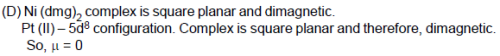QUESTION: 27

Which of the following complexes shows ionisation as well as hydrate isomerism ?

Solution: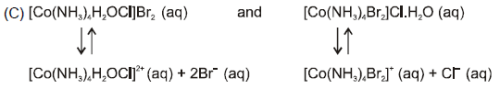Both compounds have same formula, but gives different ions in solution, hence exhibit, ionization isomerism. It also shows hydrate isomerism as shown above because hydrate isomers differ by whether or not a water molecule(s) is / are directly bonded to the metal ion or merely present as free water molecule(s) in the crystal lattice.

QUESTION: 28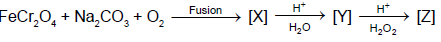Which of  the following statements is true for the compounds [X], [Y] and [Z] ?

Solution: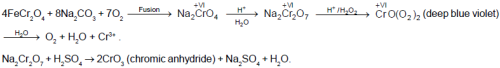QUESTION: 29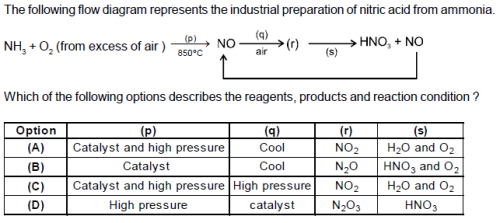Solution: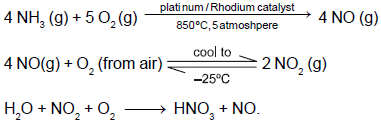QUESTION: 30

Maximum number of electrons in a subshell with = 3 and = 4 is

Solution:
QUESTION: 31

When Cl2 water in excess is added to a salt solution containing chloroform layer turns pale yellow. Salt contains :

Solution: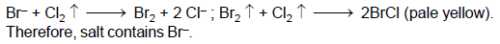QUESTION: 32

A metal nitrate solution reacts with dilute hydrochloric acid to give a white precipitate which is soluble in concentrated potassium chloride. White precipitate on passing of hydrogen sulphide gas is converted into black precipitate. The black precipitate on boiling with hydrogen peroxide (3%) is converted again to a white precipitate which is soluble in ammonium acetate. The cation of the metal nitrate is :

Solution: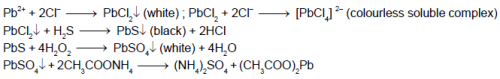*Multiple options can be correct
QUESTION: 33

Which of the following statements is(are) true for [Cu(NO2)6]4– and [Fe(H2O)6]3+ ?

Solution: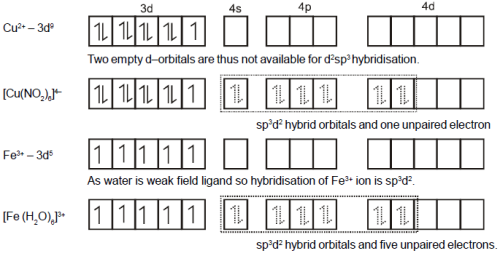*Multiple options can be correct
QUESTION: 34

KI solution is the reagent for :

Solution: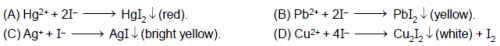*Multiple options can be correct
QUESTION: 35

The products of the reaction between one mole of XeO3 and two mole of XeF6 is(are) :

Solution: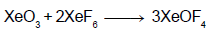QUESTION: 36

The energy of an electron in first Bohr orbit of H-atom is –13.6 eV. The possible energy value of electron in the excited state of Li2+ is

Solution:
QUESTION: 37

Statement-1 : Cuprous ion (Cu+) is colourless whereas cupric ion (Cu2+) produces blue colour in solution.

Statement-2 : Cuprous ion (Cu+) has unpaired electrons while cupric ion (Cu2+) does not.

Solution:

Cupric ion produces blue colour in solution due to the presence of unpaired electron (d-d transition) while cuprous ion does not have unpaired electron.

QUESTION: 38

Statement-1 : In the cyanide process for the extraction of gold and silver from their native ores, the cyanide solution acts as a reducing agent to reduce the gold and silver compounds present in the ores into the metallic states.

Statement-2 : In the extraction of gold and silver, the cyanide solution acts as  complexing reagent in the presence of air and form their respective soluble complexes.

Solution:

S-1 :  Cyanide solution acts as leaching agent. This dissolves the ores of silver and gold forming soluble complexes. It does not convert ores into directly metallic state. So S-1 is false.

S-2 : True statement.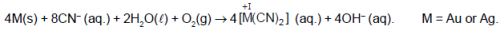QUESTION: 39

Four wires of identical lengths, diameters and material and stretched on a sonometer box. The ratio of their tension is 1: 4: 9: 16. the ratio of their fundamental frequencies is

Solution:
QUESTION: 40

A wave is propagating along X-axis and another identical wave is propagating along Y-axis if they superimpose each other, the resultant wave will be

Solution:
QUESTION: 41

What is the maximum numbers of electrons that can be associated with the following set of quantum numbers? [NEET 2013]

n = 3, = 1 and m = –1

Solution:
QUESTION: 42

In allene (C3H4), the type(s) of hybridisation of the carbon atoms is (are)

Solution:
QUESTION: 43

In Bohr series of lines of hydrogen spectrum, the third line from the red end corresponds to which one of the following inner-orbit jumps of the electron for Bohr orbits in an atom of hydrogen?

Solution:
QUESTION: 44

An electron is moving in Bohr's fourth orbit. Its de-Broglie wavelength is λ. What is the circumference of the fourth orbit?

Solution:
QUESTION: 45

Two particles C and D are in motion. If the wavelength of particle C is 5 x 10-8m. Calculate the wavelength of particle D if its momentum is half of C.

Solution:
QUESTION: 46

Which of the following will fail to react with potassium dichromate and dilute sulphuric acid

Solution:
QUESTION: 47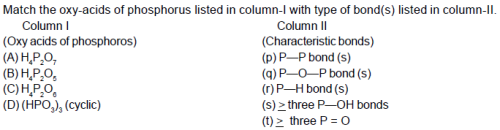Solution: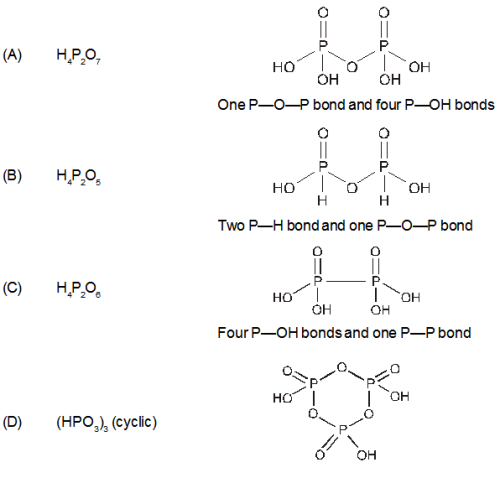QUESTION: 48

The oxidation of toluene to benzaldehyde by chromyl chloride is called

Solution:
QUESTION: 49

Hydrolysis of ozonide of 1-butene gives

Solution:
QUESTION: 50

If adj B = A, |PBQ| = 1 and |P|, |B|, |Q| belongs to set of natural numbers then (Q–1 B P–1)–1 is equal to

Solution: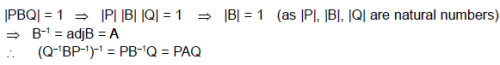QUESTION: 51

When Cl2 gas reacts with hot and concentrated sodium hydroxide solution, the oxidation number of chlorine changes from

Solution:
QUESTION: 52

Three reactions involving H2PO4are given below

(i) H3PO4 + H2O ® H3O+ + H2PO4-

(ii) H2PO4- + H2O ® HPO42- + H3O+

(iii) H2PO4- + OH- ® H3PO4 + O2-

In which of the above does H2PO4- act as an acid?

Solution:
QUESTION: 53

Which one of the following arrangements represents the correct order of least negative to most negative electron gain enthalpy for C, Ca, Al, F and O?

Solution:
QUESTION: 54

Roasting of sulphides gives the gas X as a byproduct This is a colorless gas with choking smell of burnt sulphur and causes great damage to respiratory organs as a result of acid rain. Its aqueous solution is acidic acts as a reducing agent and its acid has never been isolated. The gas X is

Solution:
QUESTION: 55

Consider the following reaction

C2H5OH + H2SO4  → Product

Among the following, which one cannot be formed as a product under any conditions?

Solution:
QUESTION: 56

In 1L saturated solution of AgCl[KSP(AgCl) = 1.6 × 10–10], 0.1 mol of CuCl [KSP(CuCl) = 1.0 × 10–6] is added.

The resultant concentration of Ag+ in the solution is 1.6 × 10–x. The value of "x" is.

Solution:
QUESTION: 57

Solubility product of silver bromide is 5.0 × 10–13. The quantity of potassium bromide (molar mass taken as 120 g mol–1) to be added to 1 litre of 0.05 M solution of silver nitrate to start the precipitation of AgBr is

Solution:
*Multiple options can be correct
QUESTION: 58

Suppose m boys and m girls take their seats randomly round a circle. The probability of their sitting is (2mCm )–1 , when

Solution: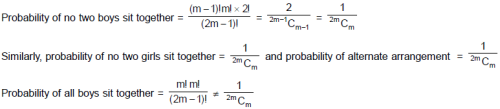*Multiple options can be correct
QUESTION: 59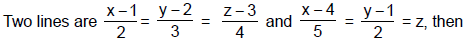Solution: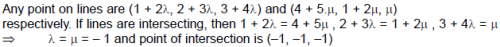*Multiple options can be correct
QUESTION: 60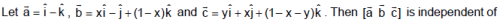Solution: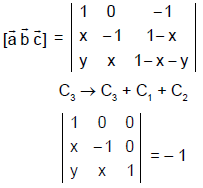QUESTION: 61

At 25°C, the solubility product of Mg(OH)2 is 1.0 × 10–11. At which pH, will Mg2+ ions start precipitating in the form of Mg(OH)2 from a

solution of 0.001 M Mg2+ ions?

Solution:
QUESTION: 62

Statement 1 : The locus represented by xy + yz = 0 is a pair of perpendicular planes.

Statement 2 : If a1x + b1y + c1z + d1 = 0 and a2x + b2y + c2z + d2 = 0 are perpendicular then a1a2 + b1b2 + c1c2 = 1.

Solution: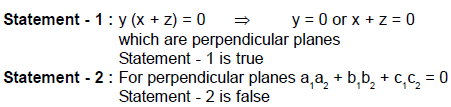QUESTION: 63

In view of the signs of ΔrG° for the following reactions

PbO2 + Pb → 2 PbO, ΔrG° < 0

SnO2 + Sn → 2 SnO, ΔrG° > 0,

Which oxidation states are more characteristic for lead and tin?

Solution:
QUESTION: 64

For a particular reversible reaction at temperature T, ΔH and ΔS were found to be both +ve. If Te is the temperature at equilibrium, the reaction would be spontaneous when

Solution:
QUESTION: 65

The work done when two mole of an ideal gas is compressed from a volume of 5 m3 to 1 dm3 at 300 K, under a pressure of 100 kPa is

Solution:
QUESTION: 66

Among the following sets of reactants which one produces anisole?

Solution:
QUESTION: 67

The electrons, identified by quantum numbers n and l
(i) n = 4, l = 1, (ii) n = 4, l = 0, (iii) n = 3, l = 2, (iv) n = 3, l = 1
can be placed in order of increasing energy, from the lowest to highest, as:

Solution:
QUESTION: 68

An objective test contains two sections : A and B, each consisting of 10 questions. In section A, only one choice out of 4 choices is correct and student is awarded 1 mark for every correct answer. In section B, one or more than one choice is (are) correct out of 4 choices and the student is awarded 3 marks if he(she) ticks only the correct choices and all the correct choices. There is no negative marking.

Q. In how many ways can a student answer to any question of section B

Solution: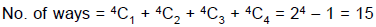QUESTION: 69

An electronic transition in hydrogen atom results in the formation of Ha line of hydrogen in Lyman series, the energies associated with the electron in each of the orbits involved in the transition (in kcal mol–1) are

Solution:
QUESTION: 70

Hybridization of C2 and C3 of

H3C – CH = C = CH – CH3 are

Solution:
QUESTION: 71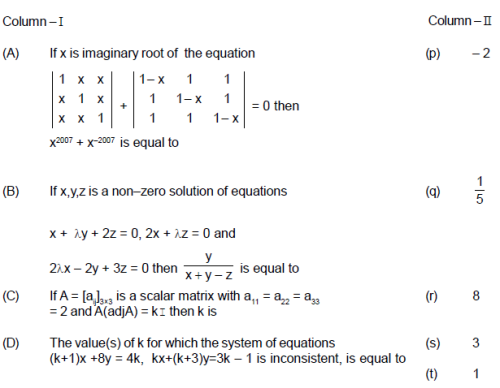Solution: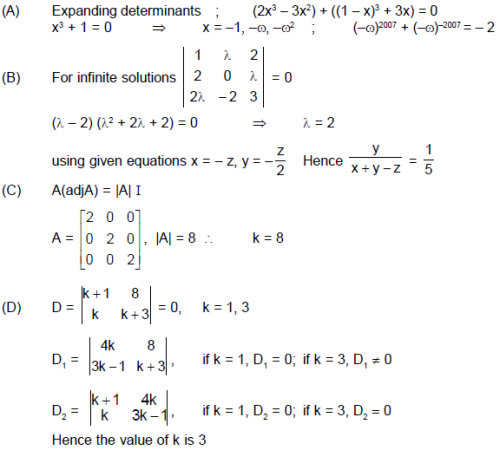QUESTION: 72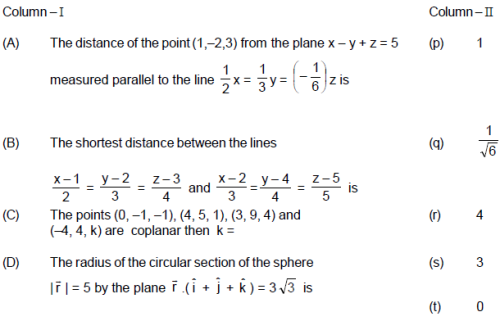Solution: